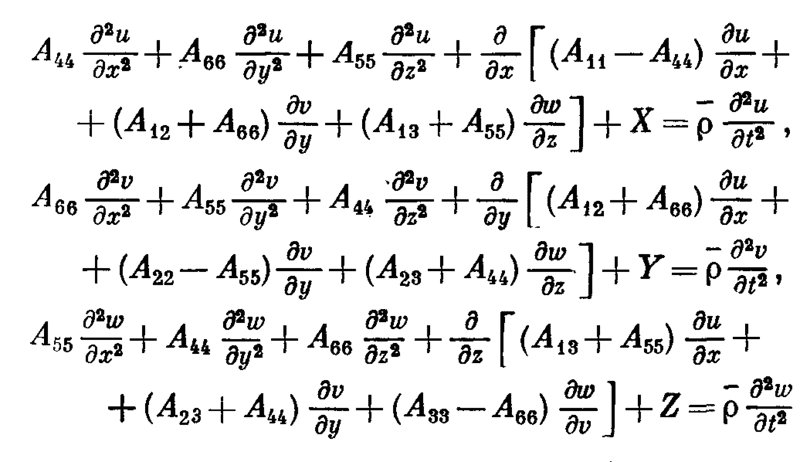# Homework help: anisotropic elasticity equations

## Homework Statement

Hello, I'm studying anisotropic elasticity. One of the books I'm using is Lekhnitskii's. The book presents the general equations of the theory of elasticity for an orthotropic body as follows:

## Homework Equations## The Attempt at a Solution

However, when I combine the strain-displacement equations and the Hook's Law and then replace them into the equilibrium equations I cannot get the same expression. My reasoning looks like this (for example, the first equation of the system):

A11.Uxx + A12.Vyx + A13.Wzx + A66.(Uyy + Vyx) + A55.(Wzx + Uzz) = 0

Obviously, I'm doing something wrong. What is my mistake?

Many thanks for your kind help.